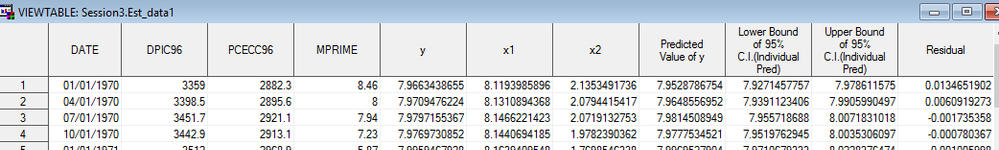## How do I get a column of observation id?

I need x to = 1 - 178 (observations). So far my commands are:

/* transform consumption and income variables into logs*/

data session3.combinedfiles;
set session3.combinedfiles;

y=log(PCECC96);
x1=log(DPIC96);
x2=log(MPRIME);
run;

/* multiple regression model: suppose Y now depends on x1 and x2: Y = beta0+ beta1 x1 + beta2 x2 + error*/

proc reg data=session3.combinedfiles;
model y = x1 x2;
output out=session3.est_data1 residual=e predicted=y_hat lcl=lower95 ucl=upper95;
run;
quit;

As you can see I don't have a column called X which simply equals id. Thank you for the help.3 REPLIES 3

## Re: How do I get a column of observation id?

Hi:
This doesn't look like a Graph question. Perhaps you would get more responses if you posted this in one of the other forums, such as the SAS Statistical Procedures forum, under Analytics.

cynthia

## Re: How do I get a column of observation id?

Thank you. I posted it there.

## Re: How do I get a column of observation id?

I'm not sure about the statistical proc, but if you just want to add an 'x' variable to your dataset, that contains the observation id, maybe something like this will work ...

data session3.combinedfiles;
set session3.combinedfiles;

x=_n_;

y=log(PCECC96);
x1=log(DPIC96);
x2=log(MPRIME);
run;

Discussion stats
• 3 replies
• 819 views
• 0 likes
• 3 in conversation